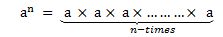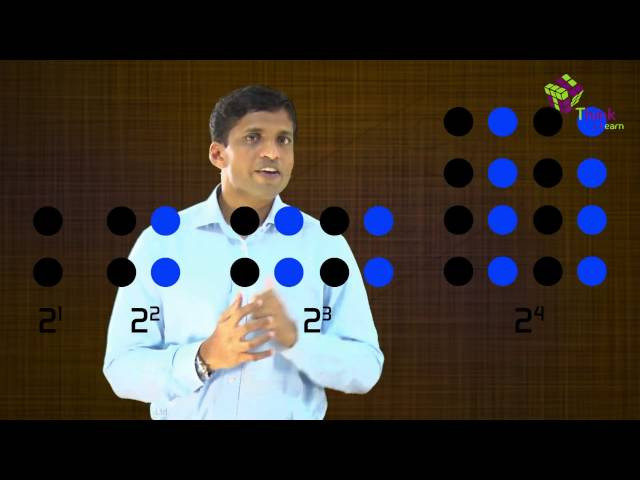# Exponents And Powers

Exponents and powers are ways used to represent very large numbers or very small numbers in a simplified manner. For example, if we have to show 3 x 3 x 3 x 3 in a simple way, then we can write it as 34, where 4 is the exponent and 3 is the base. The whole expression 34 is said to be power. Also learn the laws of exponents here.

Basically, power is an expression that shows repeated multiplication of the same number or factor. The value of the exponent is based on the number of times the base is multiplied to itself. See of the examples here:

 22 = 2 raised to power 2 = 2 x 2 = 4 53 = 5 raised to power 3 = 5 x 5 x 5 = 125

## What is Exponent?

An exponent of a number, represents the number of times the number is multiplied to itself. If 8 is multiplied by itself for n times, then, it is represented as:

8 x 8 x 8 x 8 x …..n times = 8n

The above expression, 8n, is said as 8 raised to the power n. Therefore, exponents are also called power or sometimes indices.

Examples:

• 2 x 2 x 2 x 2 = 24
• 5 x 5 x 5 = 53
• 10 x 10 x 10 x 10 x 10 x 10 = 106

### General Form of Exponents

The exponent is a simple but powerful tool. It tells us how many times a number should be multiplied by itself to get the desired result. Thus any number ‘a’ raised to power ‘n’ can be expressed as:Here a is any number and n is a natural number.

an is also called the nth power of a.

‘a’ is the base and ‘n’ is the exponent or index or power.

‘a’ is multiplied ‘n’ times, and thereby exponentiation is the shorthand method of repeated multiplication.

## Laws of Exponents

The laws of exponents are demonstrated based on the powers they carry.

• Bases – multiplying the like ones – add the exponents and keep the base same. (Multiplication Law)
• Bases – raise it with power to another – multiply the exponents and keep the base same.
• Bases – dividing the like ones – ‘Numerator Exponent – Denominator Exponent’ and keep the base same. (Division Law)

Let ‘a’ is any number or integer (positive or negative) and ‘m’,  ‘n’ are positive integers, denoting the power to the bases, then;

### Multiplication Law

As per the multiplication law of exponents, the product of two exponents with the same base and different powers equals to base raised to the sum of the two powers or integers.

am × an  = am+n

### Division Law

When two exponents having same bases and different powers are divided, then it results in base raised to the difference between the two powers.

am ÷ an  = am / an  = am-n

### Negative Exponent Law

Any base if has a negative power, then it results in reciprocal but with positive power or integer to the base.

a-m  = 1/am

## Rules of Exponents

The rules of exponents are followed by the laws. Let us have a look at them with a brief explanation.

Suppose ‘a’ & ‘b’ are the integers and ‘m’ & ‘n’ are the values for powers, then the rules for exponents and powers are given by:

i) a0 = 1

As per this rule, if the power of any integer is zero, then the resulted output will be unity or one.

Example: 50 = 1

ii) (am)n = a(mn)

‘a’ raised to the power ‘m’ raised to the power ‘n’ is equal to ‘a’ raised to the power product of ‘m’ and ‘n’.

Example: (52)= 52 x 3

iii) am × bm =(ab)m

The product of ‘a’ raised to the power of ‘m’ and ‘b’ raised to the power ‘m’ is equal to the product of ‘a’ and ‘b’ whole raised to the power ‘m’.

Example: 52 × 62 =(5 x 6)2

iv) am/bm = (a/b)m

The division of ‘a’ raised to the power ‘m’ and ‘b’ raised to the power ‘m’ is equal to the division of ‘a’ by ‘b’ whole raised to the power ‘m’.

Example: 52/62 = (5/6)2

## Solved Questions

Example 1: Write 7 x 7 x 7 x 7 x 7 x 7 x 7 x 7 in exponent form.

Solution:

In this problem 7s are written 8 times, so the problem can be rewritten as an exponent of 8.

7 x 7 x 7 x 7 x 7 x 7 x 7 x 7 = 78.

Example 2: Write below problems like exponents:

1. 3 x 3 x 3 x 3 x 3 x 3
2. 7 x 7 x 7 x 7 x 7
3. 10 x 10 x 10 x 10 x 10 x 10 x 10

Solution:

1. 3 x 3 x 3 x 3 x 3 x 3 = 36
2. 7 x 7 x 7 x 7 x 7 = 75
3. 10 x 10 x 10 x 10 x 10 x 10 x 10 = 107

Example 3: Simplify 253/53

Solution:

Using Law: am/bm = (a/b)m

253/53  can be written as (25/5)3  = 53 = 125.

## Exponents and Powers Applications

Scientific notation uses the power of ten expressed as exponents, so we need a little background before we can jump in. In this concept, we round out your knowledge of exponents, which we studied in previous classes.

The distance between the Sun and the Earth is 149,600,000 kilometres. The mass of the Sun is 1,989,000,000,000,000,000,000,000,000,000 kilograms. The age of the Earth is 4,550,000,000 years. These numbers are way too large or small to memorize in this way.  With the help of exponents and powers, these huge numbers can be reduced to a very compact form and can be easily expressed in powers of 10.

Now, coming back to the examples we mentioned above, we can express the distance between the Sun and the Earth with the help of exponents and powers as following:

Distance between the Sun and the Earth 149,600,000 = 1.496× 10 × 10 × 10 × 10 × 10× 10 × 10 = 1.496× 108 kilometers.

Mass of the Sun: 1,989,000,000,000,000,000,000,000,000,000 kilograms = 1.989 × 1030 kilograms.

Age of the Earth:  4,550,000,000 years = 4. 55× 109 years

## Video LessonThis section covers the few important concepts related to powers and exponents. For any further query on this topic install BYJU’S – The Learning App and excel your knowledge.

## Frequently Asked Questions – FAQs

Q1

### What are powers and exponents?

Power is an expression that represents the repeated multiplication of value or integer. In general, an is a power where a is the base and n is the exponent.
For example, 63 is the power which shows that 6 is multiplied by itself three times.
Q2

### Are Powers and exponents the same?

If N is an integer and is raised to another integer, say ‘a’, then the whole expression, N^a, is the power and a is the exponent.
Q3

### What are the examples of exponents?

The examples of exponents are:
2 x 2x 2 =23 (2 raised to 3rd power)
5x5x5x5 = 54 (5 raised to 4th power)
9x9x9x9x9 = 95 (9 raised to 5th power)
Q4

### What are the rules of exponents?

The rules of exponents are:
a0 = 1
am an = am+n
am/an = am-n
(am)n = amn
Q5

### What are negative exponents?

A negative exponent is used when 1 is divided by repeated multiplication of a factor. Say, 1/n is given by n-1, where -1 is the exponent. If a number is raised to negative exponents then it represents the reciprocal of it. For example, 3 raised to -2 is represented by 3-2, which is equal to 1/32.
Q6

### What do we get if the exponent is 1 or 0?

If the exponent of a base number is one, then the value of the base remains unchanged. For example, 91 = 9
If the exponent is 0, then we get 1 as the final result. For example, 90 = 1